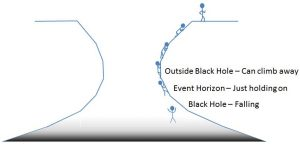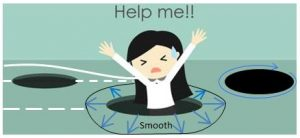By James Lawless, University of Wollongong

The aim of this project was to learn what the area theorem for a black hole is and why it is an open problem.

A black hole is a region in 3D, like a ball, that once you enter you cannot leave and the event horizon is the boundary of this region, the surface of the ball. Figure 1 gives an analogy for the black hole and event horizon in 2D.Figure 1. Rock climbing analogy for a black hole

The area theorem for a black hole states that “the surface area of the event horizon will only increase as time passes by.” The project looked at a proof for the area theorem that makes an extra assumption about the event horizon. The assumption requires the event horizon to be smooth. The assumption of a smooth surface rules out many types of black holes; merging black holes, hungry black holes (eating stars!) and rotating black holes.

In Figure 2 we see what smoothness means. At each point on the event horizon (the edge of the hole in the picture) there is a unique way to pick an outward pointing perpendicular. For rotating black holes there are “cusps’’ at the poles like on an apple, and that prevents us being able to choose a perpendicular in a continuous way.Figure 2. Types of black holes 

The open question is to prove the area theorem for a more realistic black hole that has a surface that is not completely smooth.

In order to come to an understanding of the area theorem over the course of the project, time was spent learning differential geometry. Differential geometry is an area of mathematics that breaks down larger geometrical objects (Earth/sphere) into very small sections (flat ground where you are standing). Lots of new notation caused a headache in the early stages. As one of my lecturers noted, “Differential geometry is that part of mathematics invariant under change of notation.”

The project applied differential geometry to black holes (very interesting! Not sure how useful to the everyday man on Earth?). Despite whether black holes are useful or not, learning mathematics in the context of black holes is fun.

The applications of differential geometry definitely extend beyond black holes. Differential geometry is applied to the study of fluid flow and forces. Most interestingly, forces (gravitational and electromagnetic) can be related to more general notions of curvature. In general relativity curvature gives the shape of Einstein’s space-time, and this curvature is the gravitational force which determines the motion of particles and light.

It turns out that the event horizon of a black hole evolves in time by following the same paths that light does. The hope for future projects is that we can prove the area theorem just by studying the behaviour of light rays near the black hole without requiring any smoothness. This would allow us to understand the evolution of complicated scenarios involving black holes, such as multiple black holes, black hole mergers and perhaps even the process of collapse.

References

 Aeaechan #1 Royalty Free Photos, Pictures, Images And Stock Photography. (n.d.). Retrieved from https://www.123rf.com/profile_aeaechan

James Lawless was a recipient of a 2018/19 AMSI Vacation Research Scholarship.